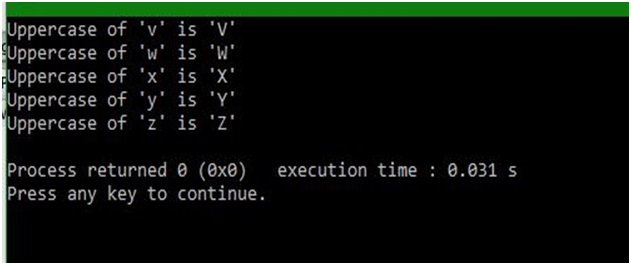# toupper() function of ctype.h in C

In this article, we are going to learn about the toupper() function of ctype.h header file in C programming language, and use it to convert the lowercase variables in uppercase.
Submitted by Abhishek Sharma, on April 11, 2018

This function is used to make a lower-case character into the upper-case character. This function takes a character in the form of a parameter as a character and returns a character. The Character which will return will be in upper-case.

This is rather useful function and makes coding simple. This can be used in different scenario, like printing the output in the particular format irrespective of the user’s input.

Anyway you can implement your own function if you don’t want to include a whole library in your program. Just check for the ascii value and modify them.

Example:

```    Input character is: 'A'
Function will return 'A'

Input character is: 'a'
Function will return 'A'
```

## ctype.h - toupper() function Example in C

﻿

```#include <stdio.h>
#include <ctype.h>

int main()
{
// defining the type of variable
char a,b,c,d,e,f,g,h,i,j;

// assigning the values of variables
a = 'v';
b = 'w';
c = 'x';
d = 'y';
e = 'z';

// getting the upper case values of the above assigned variables
f = toupper(a);
g = toupper(b);
h = toupper(c);
i = toupper(d);
j = toupper(e);

// printing the lower case and their upper case values

printf("Uppercase of '%c' is '%c'\n", a, f);

printf("Uppercase of '%c' is '%c'\n", b, g);

printf("Uppercase of '%c' is '%c'\n", c, h);

printf("Uppercase of '%c' is '%c'\n", d, i);

printf("Uppercase of '%c' is '%c'\n", e, j);

return 0;
}
```

Output